Trial ends in

# The Traceable Calibration of Thrust Stand by Electrostatic Force

Published: March 1, 2022 doi: 10.3791/63465

### Abstract

Micro thrusters have important applications in low-frequency gravitational wave detection, satellite formation, and inter-satellite laser communication, so it is necessary to accurately measure the thrust of micro thrusters with traceability. A thrust stand is a widely used micro thrust measuring device with the advantages of high resolution and large load. Traceability calibration of mechanical characteristics of thrust stand is an essential prerequisite to ensure traceability measurement of thrust. In this study, a parallel plate capacitor was used to calibrate the thrust stand by generating a micronewton electrostatic force, which could be traced to the International System of Units (SI). The constant capacitance gradient range was obtained through simulation and theoretical calculation. Moreover, the electrostatic force could be changed by standard voltage with the advantages of simple principle, instantaneous trigger, and traceability. The device could be used for traceability calibration of micro newton thrust stand due to simple assembly and short traceability path.

### Introduction

The micro thruster is indispensable for the ultra-static and ultra-stable space experimental platform to provide micro thrust to offset the non-conservative force on the spacecraft in real-time in low-frequency gravitational wave detection. Reliable measurement of the thrust of the micro thruster in the complex noise environment is the premise to achieve drag-free control. Therefore, it is essential to calibrate the thrust stand with high precision to establish the mechanical response model. The calibration methods of thrust stand mainly include two types, contact and non-contact calibration methods.

Contact calibration methods mainly include rope pulley weight system, impact hammer, and impact pendulum, which are traditional calibration methods. In 2002, Lake et al.1 used weights and pulleys to apply calibration force in the range of mN. In 2006, Polzin et al.2 also used a similar automatic system to load vertical loads into the swing arm, but it had a large error when the force was less than 10 mN. In 2004, Koizumi et al.3 obtained the generated momentum by integrating the force recorded by the force sensor in the collision process. The resolution of the force sensor was 90 mN, the effective impulse was 20-80 µNs, and the total error was 2.6 µNs at 100 µNs. The impact pendulum is only suitable for large impulse measurement because mechanical vibration seriously affects the calibration. Although the contact calibration method is easy to set up, there is zero drift error, and the calibrated force is generally larger than the non-contact methods. Therefore, it is not suitable for calibrating the micro force thrust stand.

Non-contact calibration methods mainly include gas dynamic calibration, electromagnetic calibration, and electrostatic calibration. In 2002, Jamison et al.4 developed a gas dynamic calibration technology, which generated a force range of 80 nN-1 µN, 86.2 nN thrust with 10.7% error, and 712 nN thrust with 2% error. Gas dynamic calibration technology can generate nN and sub-µN force reliably and is easy to implement. However, it is a kind of indirect calibration technology that cannot trace to the International System of Units (SI). What is more, gas dynamic calibration is only suitable in a vacuum.

The electromagnetic force can be as small as the order of micronewton, and there is a good linear relationship between the electromagnetic force and the current, which has good repeatability. Tang et al.5 developed an electromagnetic calibration technology using a permanent magnet and coil. The measurement range was 10-1000 µNs, the calibration force was less than 10 mN, and the calibration reliability of 310 µN is 95%. In 2013, He et al.6 used the ring electromagnet with air gap and the energized copper wire for calibration. The calibration uncertainty of 150 µN force was 4.17 µN, and the calibration force had a large range and was not sensitive to the displacement of the thrust stand arm, but there was a problem that the copper wire current would magnetize the electromagnet core. In 2019, Lam et al.7 used different magnets and commercial voice coils to calibrate a wide range of forces. The structure was compact and easy to install. Moreover, the force range was large, with four orders of magnitude of 30-23000 µN, and the uncertainties of static and pulse force were 18.47% and 11.38%, respectively. However, for the calibration of the thrust frame, the electromagnetic force is not traceable to SI.

Electrostatic force calibration is the most widely used direct calibration technique. Selden and Ketsdever8 used an electrostatic comb (ESC) as the calibration device with a measuring range of dozens of micronewton with an error of 3%. The force changed 2% as plate spacing changed 1 mm. However, the distance between the adjacent teeth should be the same, which was only applicable to the thrust stand with small displacement. In 2012, Pancotti et al.9 designed a symmetrical electrostatic comb whose pulse range was 0.01 mNs-20 mNs, which could generate a larger electrostatic pulse. However, the disadvantages of complex structure and easy damage of electrostatic comb need to be solved.

It is a prerequisite to provide the traceable micronewton force as a reference force to calibrate the thrust stand. The electrostatic force is widely used to trace force to SI in the metrology Institute10,11,12. The electrostatic force has the advantages of simple principle, instantaneous trigger, and short tracing path. In this study, the parallel plate capacitor was served to generate electrostatic force as a reference force to calibrate the pendulum thrust stand, whose displacement output is proportional to the applied thrust. The ratio of the thrust and the displacement is the stiffness of the thrust stand. By calibrating the capacitance gradient of the capacitor, it was unnecessary to strictly control the pose of two parallel plates. The constant capacitance gradient range was obtained through simulation and theoretical calculation. The range of electrostatic force could be adjusted by the spacing and area of two plates, which was suitable for efficient calibration of thrust stand with different stiffness.

Subscription Required. Please recommend JoVE to your librarian.

### Protocol

1. Experimental realization

1. Gather all system components, including the circular parallel plate capacitor, the motorized linear stage, the thrust stand, the capacitance bridge, the SMU instrument, the laser interferometer, and other components, shown in Figure 1.
2. Fix Plate A on the motorized linear stage and fix Plate B on the arm of the thrust stand, making plates A and B parallel to each other.
NOTE: The plates are processed by high precision grinding of aluminum alloy. The diameter of plate A is 6 cm, and the diameter of plate B is 4 cm so the alignment error can be ignored.
3. Control the distance Dab between the two plates by a motorized linear stage (Resolution 0.625 µm). Completely fit the two plates and then pull a fixed distance of 1 mm through the linear stage.
4. Connect the capacitance bridge (Resolution 0.8 aF, Accuracy ±5 PPM) with the two plates to measure the capacitance Cab variation with the change of plate spacing.
5. Apply a standard voltage to the capacitor by a high voltage source measure unit (SMU) instrument (Precision 0.012%, ±5 - ±1100 V) to generate a controllable high precision electrostatic force.
6. Adjust the laser interferometer (Resolution 10 nm) to directly face the arm of the thrust stand, and measure the displacement x in real-time.

2. Calibration of the capacitance gradient

1. Drive plate A to move to the side away from plate B with a step length of 0.02 mm by the motorized linear stage, and make the initial plate spacing equal to 1 mm.
2. Measure the capacitance value of the two parallel plates by the capacitance bridge after each step until the relative change of plate spacing is 0.12 mm.
3. Begin a reverse step with a length of 0.02 mm to return to the initial position.
4. Conduct a total of five repeatable experiments.
5. Fit the results to get the relationship between capacitance gradient and plate spacing of parallel plate capacitor, dCab/dDab.

3. Electrostatic force calibration of thrust stand

1. Disconnect the capacitor bridge from the parallel plate capacitor.
2. Connect the two plates with the SMU instrument and make the space between the two plates equal to 1 mm.
3. Increase the voltage U from zero step by step at both plates of the capacitor with a step value of 50 V until the applied voltage is 300 V. The electrostatic force F is equal to 1/2U2(dCab/dDab).
4. Use the laser interferometer to measure the displacement x of the thrust stand arm in real-time. Set the sampling frequency of the laser interferometer to 50 Hz.
5. Decrease the voltage U from 300 V step by step at both plates of the capacitor with a step value of 50 V until the applied voltage is zero.
6. Conduct a total of five repeatable experiments.
7. Fit the results to get the relationship between the electrostatic force F and the displacement x of the thrust stand arm. Calculate the stiffness k of the thrust stand according to Hooke's law, k = F/x.

Subscription Required. Please recommend JoVE to your librarian.

### Representative Results

Following the protocol, the capacitance gradient and the stiffness of the thrust stand are calibrated. The principle of electrostatic force should be introduced. There will be relative motion Dab between two charged plates under the action of external force F. Moreover, the work W by external force will be converted into electric energy E stored in the capacitor. The potential difference U, the charge of both plates Q and capacitance C can be obtained. The stored energy between two plates can be calculated according to Eq.(1). The work done by external force can be calculated according to Eq.(2).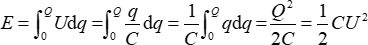(1)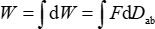(2)

Based on the principle of virtual work13,14,15, W is equal to E.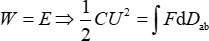(3)

The external force changes the distance dz between two plates, then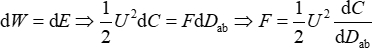(4)

Therefore, the electrostatic force can be calculated by voltage as long as the capacitance gradient is calibrated based on Eq.(4). Two circular metal plates are used to form a parallel plate capacitor.

Figure 2 shows the fitting curve of mean values of capacitance and displacement of plate A and plate B. The average of the capacitance gradient dCab/dDab is 6.2 pF/mm, and the goodness of fit can reach 0.9983. Therefore, according to Eq. (4), the parallel plate capacitor can produce traceable force less than 1 µN (as long as the voltage is less than 17.96 V), and the force value range is ~0.279 mN.

Figure 3A shows the displacement change of the thrust stand arm with the change of the electrostatic force. As the applied voltage changes equidistantly, each step displacement becomes larger and larger. It can be seen that the displacement changes symmetrically as the voltage increases and the voltage decreases. Figure 3B shows the fitting curve of mean values of the displacement of the thrust stand and the electrostatic force. The stiffness k of the thrust stand is 3.176 N/m based on Eq. (5), and the repeatability is 0.0023 N/m.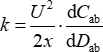(5)

The capacitance of the parallel plate capacitor can be described as Eq.(6) under the condition of ignoring the edge effect.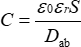(6)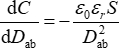(7)

S is the overlap area, the permittivity of vacuum ε0 = 8.85 x 10-12 F/m, and the relative dielectric constant εr = 1 in the air. It can be seen that the capacitance gradient changes with the plate spacing. However, the change is very small when the plate spacing changes very little. The capacitance gradient tends to a constant value. According to the request of the mN magnitude standard force for the thrust stand calibration, the plate diameter of the circular parallel plate capacitor should be within the centimeter magnitude and the distance Dab should be within the millimeter magnitude based on the Eq. (1) and (2) when the output voltage of the SMU is a few hundred volts. The output range of the SMU instrument is ±5 µV- ±1100 V. Hence, the diameters of plates A and B are 6 cm and 4 cm, respectively. The distance Dab between the two plates is 1 mm.

In order to obtain the constant capacitance gradient range, the potential distribution, electric field distribution, capacitance, and other parameters of the parallel plate capacitor with high voltage are calculated using finite element simulation. The potential distribution of parallel plate capacitors is shown in Figure 4, whose size is consistent with the actual device. In order to meet the demand of micronewton electrostatic force, the plate spacing is set to 1 mm. Figure 5 shows the electric field distribution of the parallel plate capacitor. The arrow represents the direction of the electric field, starting from the high voltage and pointing to the grounding terminal. The capacitance of the parallel plate capacitor is simulated by changing plate spacing. Step size is 20 µm and ends at 1.2 mm. As shown in Figure 6, the capacitance gradient is a constant value of 9.81 pF/mm, when the plate spacing is between 1 mm and 1.2 mm. According to Eq.(4), when the capacitance gradient is a fixed constant, the magnitude of the electrostatic force has a linear relationship with the square of the applied voltage. Figure 7 shows the simulated electrostatic force at different voltages, which conforms to the derivation of Eq. (4). When the distance between two plates is 1-1.2 mm, to produce the calibration force of 1-1000 µN, according to the simulation calculation, the voltage range is 14.29-451.57 V.

Finally, the uncertainty of calibration results is evaluated below.

1. Type A evaluation of relative uncertainty
The main sources of uncertainty are air disturbance and ground vibration. According to the Bessel method, the relative uncertainty urel(re) is 0.0724% when the mean value of the five measurements is used as the best estimate of the measurement results.

2. Type B evaluation of relative uncertainty
a. Relative uncertainty of displacement measured by the laser interferometer
The laser interferometer is used to measure the displacement of the thrust stand. The relative uncertainty of laser interferometer without compensation is 0.005%, which can be ignored.

b. Relative uncertainty of voltage applied by SMU instrument
The relative uncertainty of the SMU instrument is 0.012%. Therefore, the relative uncertainty introduced by applied voltage urel(U) is 0.012%.

c. Relative uncertainty of capacitance gradient dCab/dDab
The capacitance gradient dCab/dDab is obtained by fitting the capacitance measured by the capacitor bridge with the distance moved by the motorized linear stage. The capacitive bridge has an accuracy of 5 PPM, whose uncertainty can be ignored. The displacement resolution of the motorized linear stage is 5 µm. As a result, the relative uncertainty of dCab/dDab is 0.16%.

3. Expanded uncertainty
The uncertainty components are independent of each other, summarized in Table 1. The relative uncertainty is 0.18%, so the standard uncertainty is 0.0057 N/m. The relative uncertainty introduced by capacitance gradient contributes most. The coverage factor is 3, so the extended uncertainty is 0.0171N /m.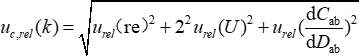(8)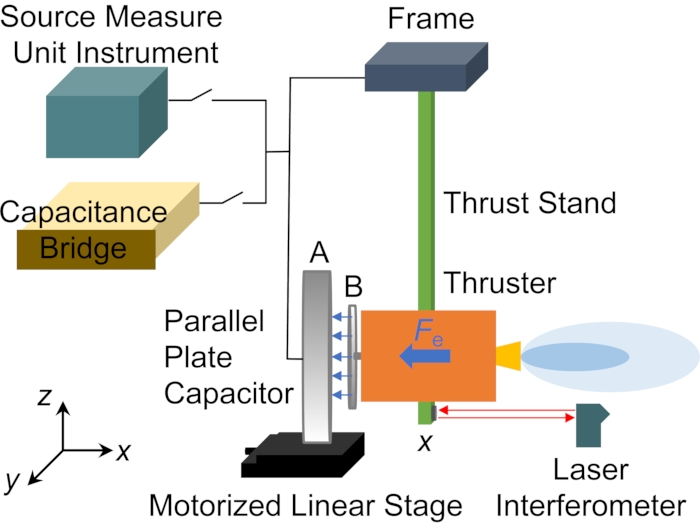Figure 1: Schematic diagram of parallel plate capacitor calibrating thrust stand. The parallel plate capacitor generated the electrostatic force as a reference for the calibration of the thrust stand. Please click here to view a larger version of this figure.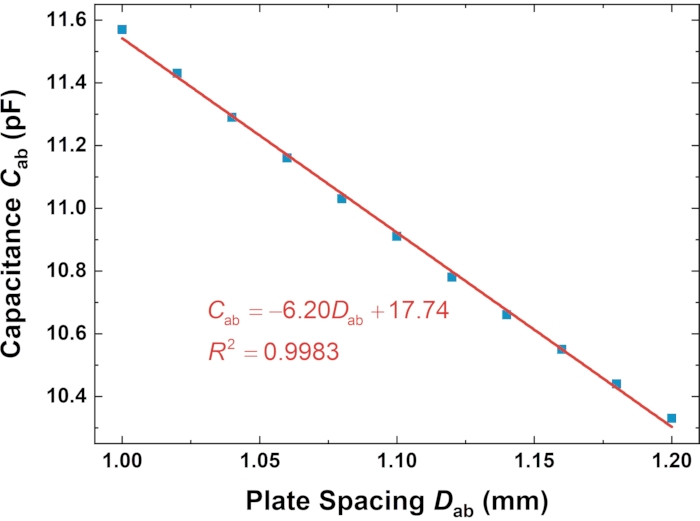Figure 2: Relationship between capacitance and plate spacing of parallel plate capacitor. The slope of the fitting line was the capacitance gradient. Please click here to view a larger version of this figure.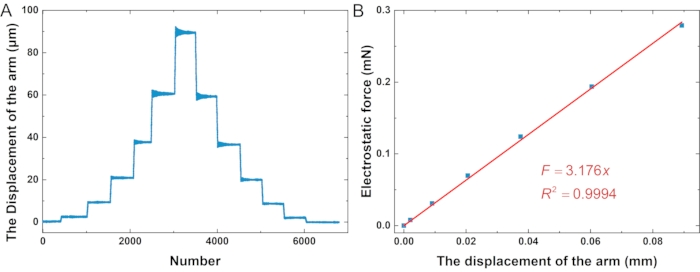Figure 3: Electrostatic force calibrating the thrust stand. (A) The displacement of the thrust stand arm. (B) The relationship between electrostatic force and displacement of the thrust stand arm. Please click here to view a larger version of this figure.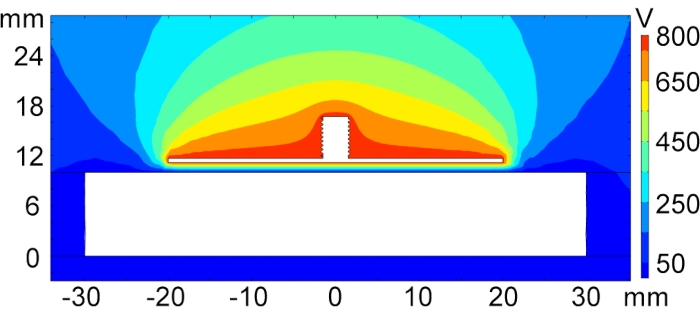Figure 4: Potential distribution of parallel plate capacitor. The Potential distribution was uniform. Please click here to view a larger version of this figure.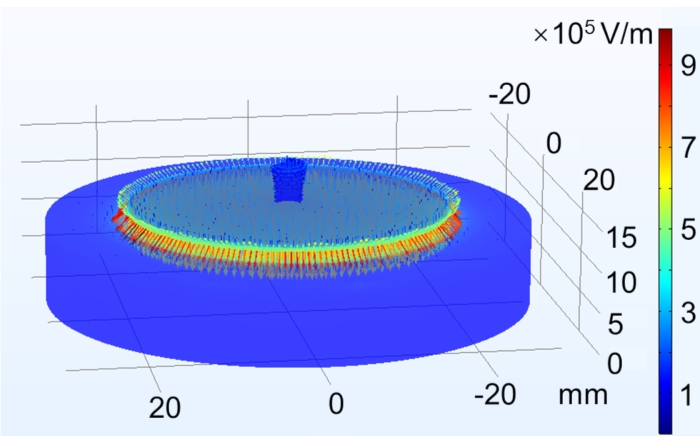Figure 5: Electric field distribution in parallel plate capacitor. The arrows represent the direction of the electric field. Please click here to view a larger version of this figure.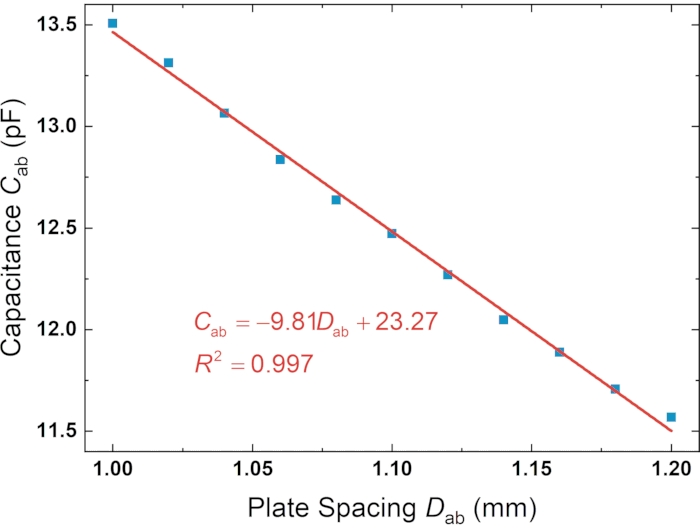Figure 6: Simulation of capacitance variation with plate spacing. The capacitance decreased with the plate spacing. Please click here to view a larger version of this figure.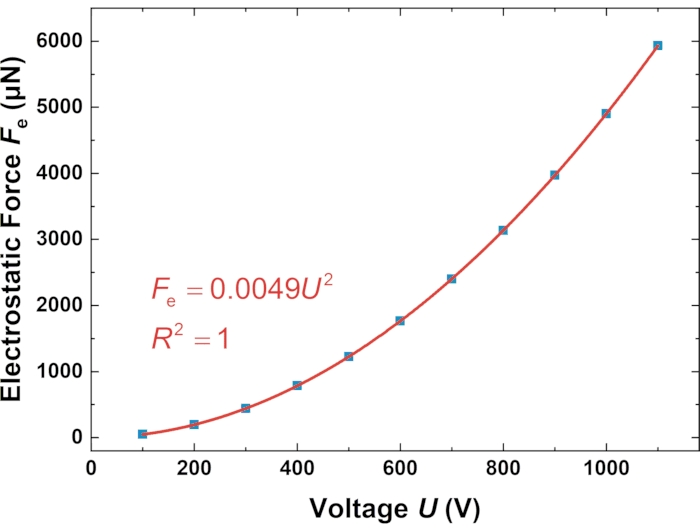Figure 7: Simulation of electrostatic force with voltage. The electrostatic force increased with the voltage applied to the plate capacitor. Please click here to view a larger version of this figure.

 Uncertainty Source Contribution to total uncertainty (%) Repeatability 0.07% U 0.01% dCab/dDab 0.16% Combined relative uncertainity 0.18%

Table 1: Uncertainty of thrust stand calibration.

Subscription Required. Please recommend JoVE to your librarian.

### Discussion

In this protocol, a parallel plate capacitor was used to calibrate the thrust stand by generating a micro-newton electrostatic force, which could be traced to SI. It is critical for all steps to calibrate the capacitance gradient precisely. The motorized linear stage made the initial plate spacing of this parallel plate capacitor equal to 1 mm and moved the plate A at a step of 0.02 mm. The capacitance bridge was used to measure the capacitance for accurately calibrating the capacitance gradient. The electrostatic force can be calculated by voltage as long as the capacitance gradient is calibrated based on Eq.(4).

The parallel plate capacitor for generating the electrostatic force in this protocol is limited by a small range. Too high a voltage is difficult to keep constant. Hence, the electrostatic force is merely suitable for calibrating the micro thrust stand.

The parallel plate capacitor had the advantages of simple principle, instantaneous trigger, and traceability. The linear response range of the parallel plate capacitor was obtained through simulation and theoretical calculation, which made it suitable for the mechanical response calibration of the thrust stand. The reference force adjusted by the voltage and the displacement measured by the laser interferometer were obtained to complete the calibration of the thrust stand.

The traceable calibration of the thrust stand based on the electrostatic force generated by the parallel plate capacitor could be less than 1 µN in a range of ~0.279 mN, with the advantages of simple assembly and short traceable path. The force measured by the thrust stand was traced to the SI traceable electrostatic force to improve the measurement accuracy. This device has a positive influence on the measurement of micro thrust as well as the design and development of micronewton thruster.

Subscription Required. Please recommend JoVE to your librarian.

### Disclosures

The authors have nothing to disclose.

### Acknowledgments

We thank the National Natural Science Foundation of China (Grant No. 11772202) for funding this work.

### Materials

 Name Company Catalog Number Comments Motorized linear stage Zolix TSA50-C Resolution 0.625 μm Capacitance bridge Andeen-Hagerling AH2550A Resolution 0.8 aF, Accuracy ±5 PPM High voltage source measure unit (SMU) instrument Keithley 2410 Precision 0.012%, ±5 μV– ±1100 V Laser interferometer Renishaw RLE10 Resolution 10 nm Circular parallel plate capacitor Processed by high precision grinding The plates are processed by high precision grinding of aluminum alloy. The diameter of plate A is 6 cm, and the diameter of plate B is 4 cm. Thrust stand Processed by high precision grinding Pendulum type thrust stand

Video Coming Soon
DOIX